Back

## Getting Lines Crossed

If this pattern continued, how many more lines of numbers would we need to add to see two lines intersect on a circled number?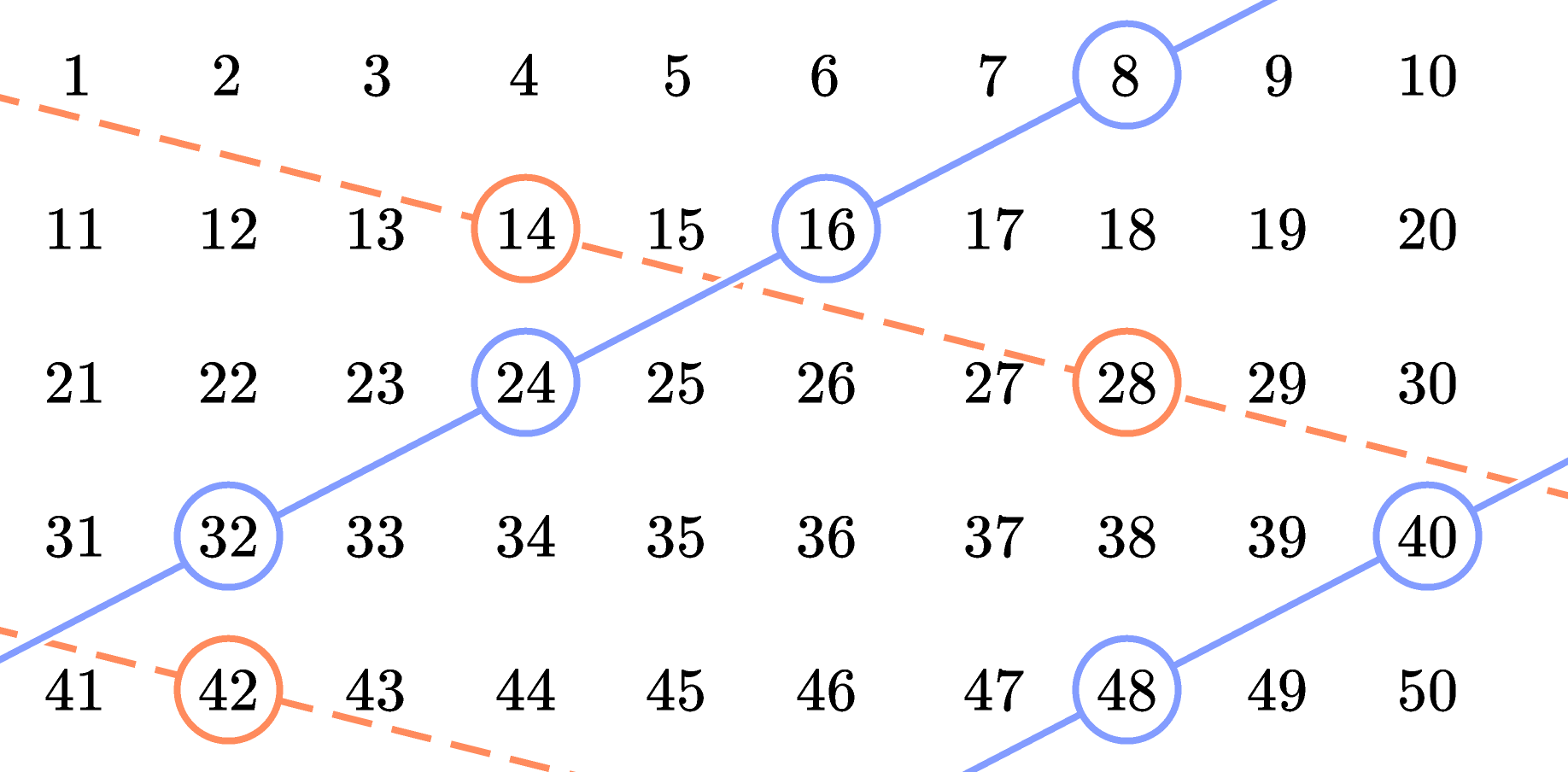Keep reading to find out, or jump ahead to the challenge for a tougher question.

To answer this question, we need to unravel any patterns in the figure. Breaking the figure apart a few pieces at a time might help, so let's start with the solid lines and the circled numbers they pass through.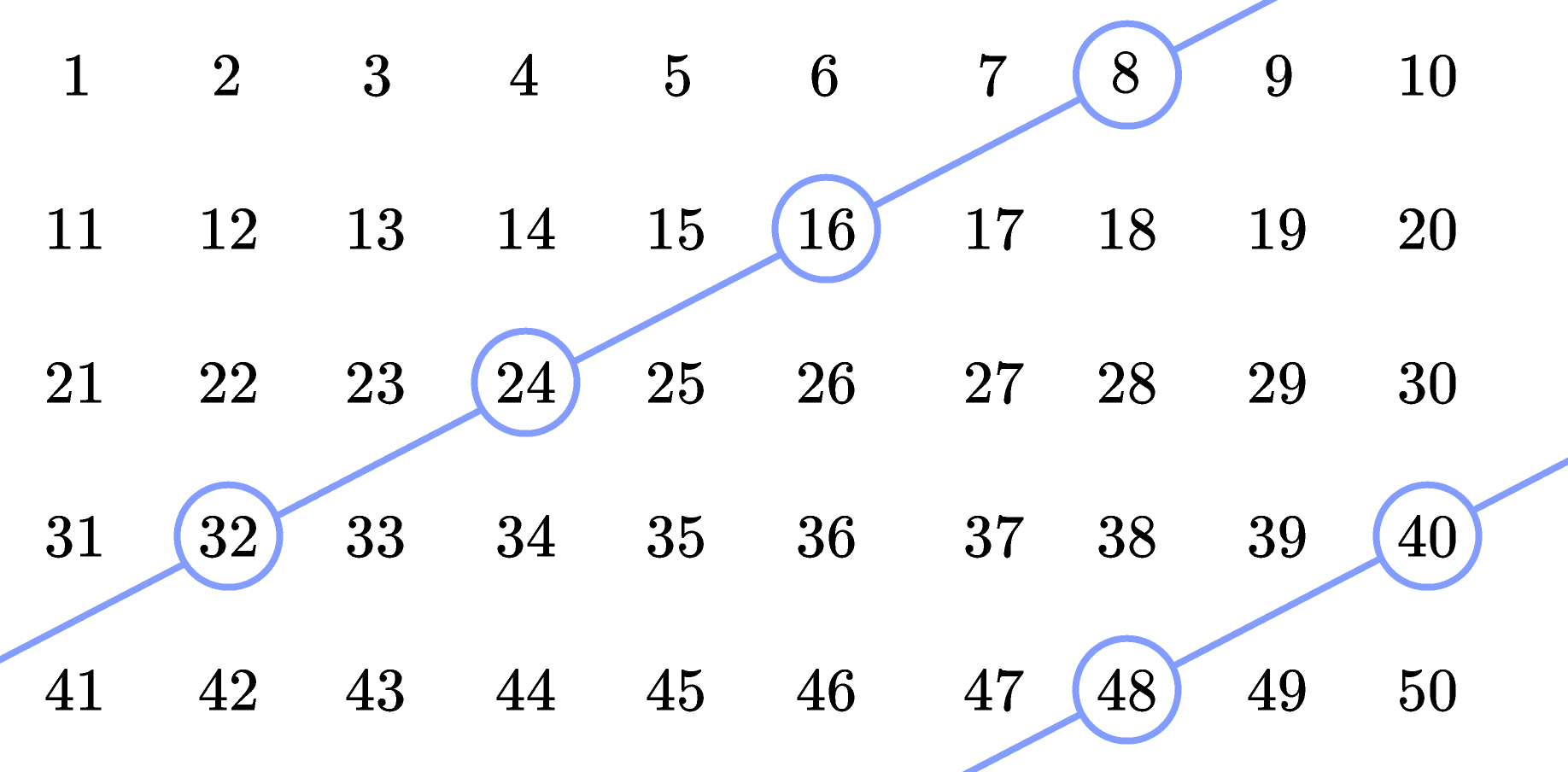Every circled number is a multiple of eight, and there aren't any uncircled multiples of eight in the figure. If we added another row of numbers, the next circled number should be $7 \times 8 = 56.$ Extending one of the solid lines takes us right through $56:$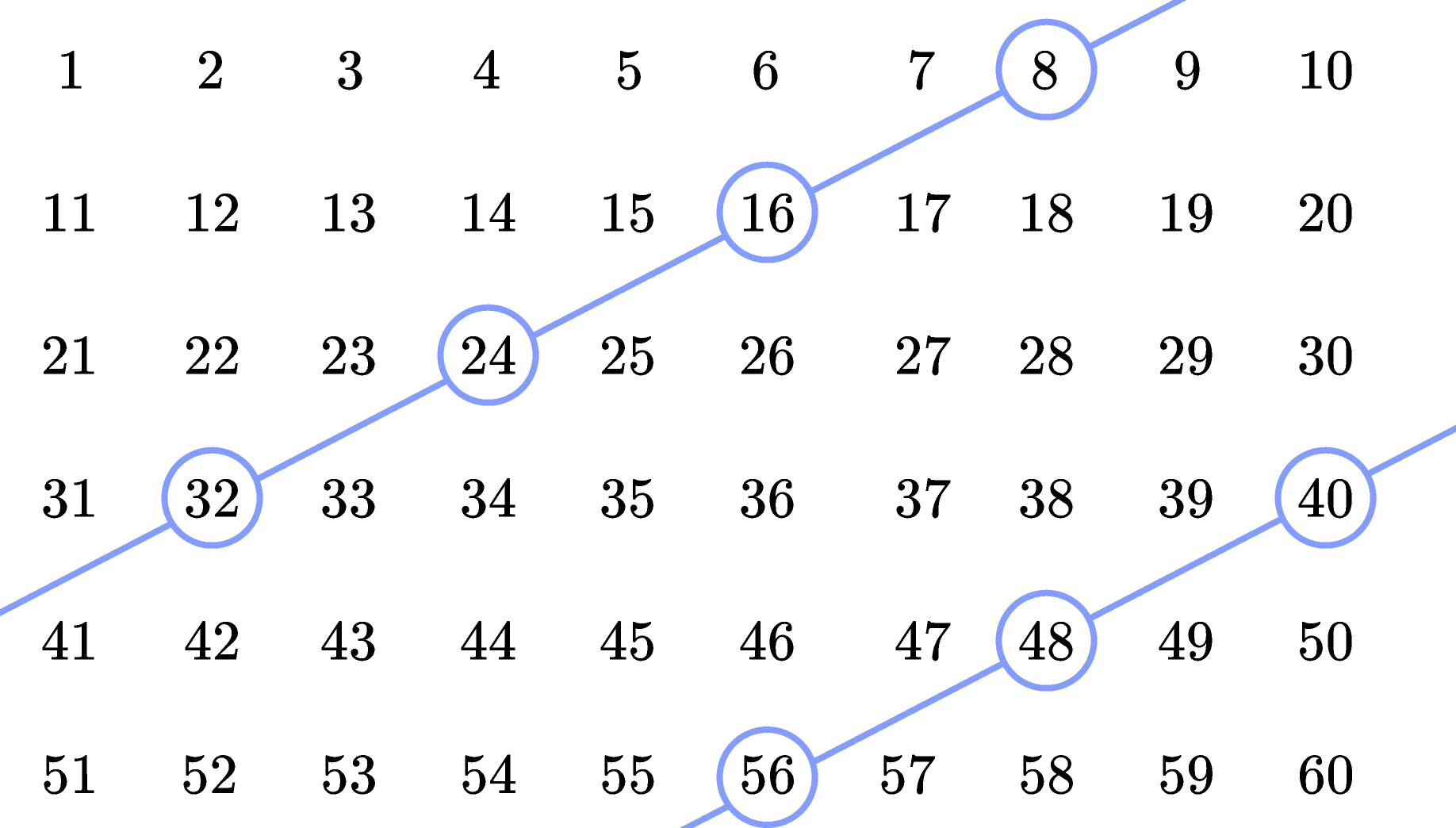Now, let's focus on the dashed lines and the circled numbers they go through. Before we added another row of numbers, the dashed lines only passed through three circled numbers. Adding the $56$ gives us a fourth circled number we can extend a dashed line through — this is because the dashed lines go through all multiples of $14:$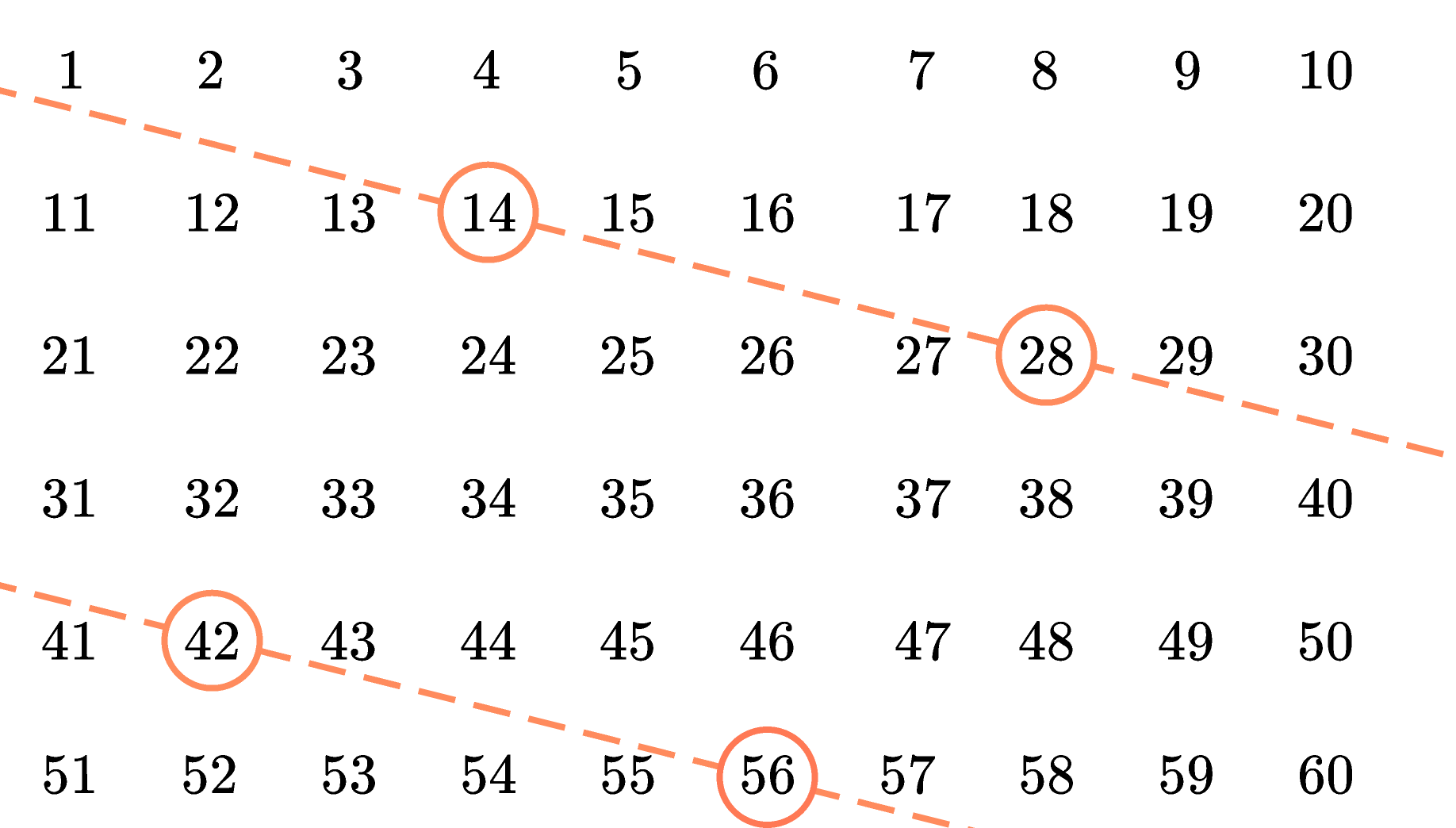The first circled number both kinds of lines go through is $56,$ since it is the least common multiple of $8$ and $14:$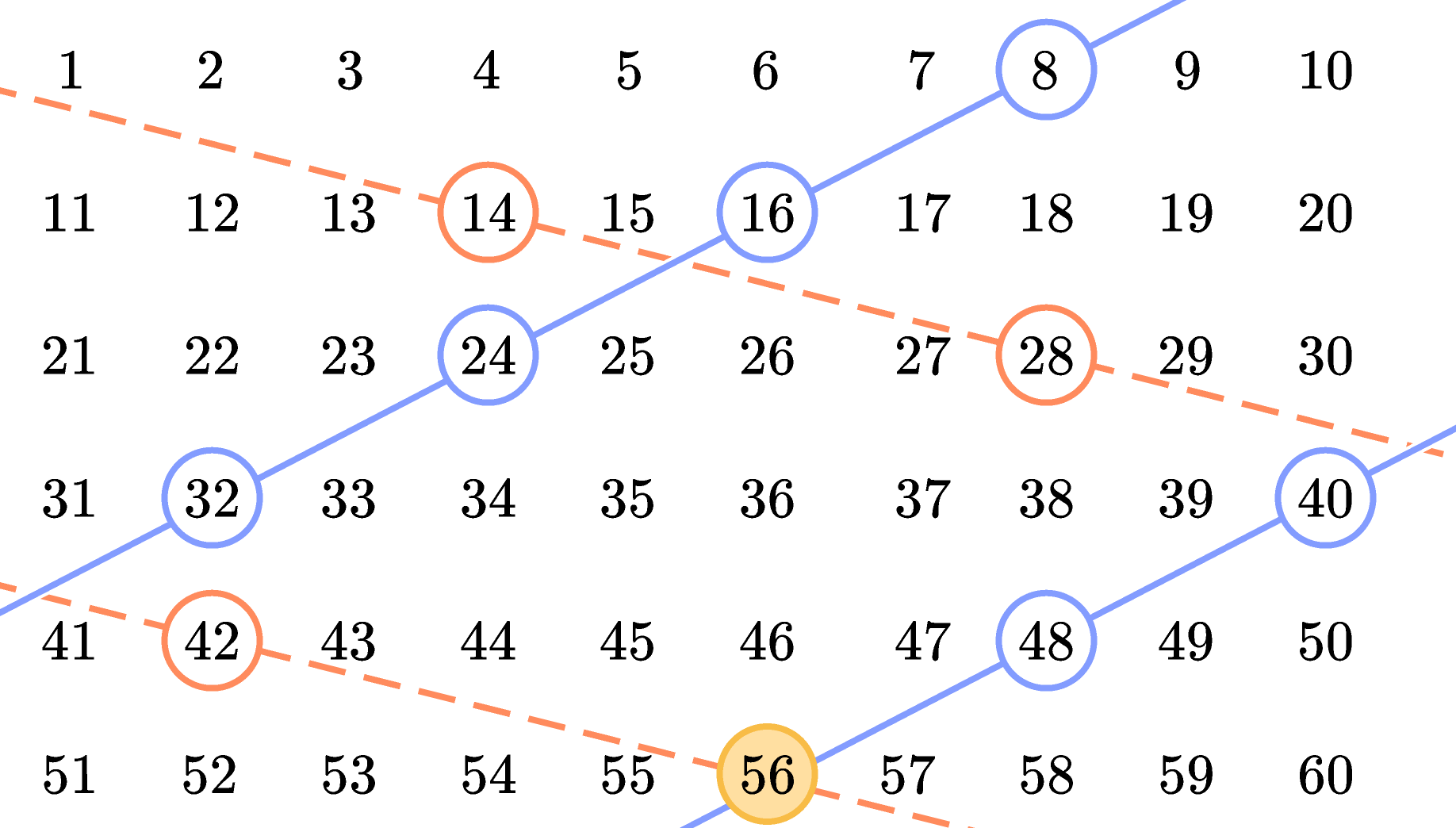What if we went all the way to $500?$ How many times would lines intersect on circled numbers?

# Today's Challenge

If this pattern continued up to the number $500,$ how many times would lines intersect on circled numbers?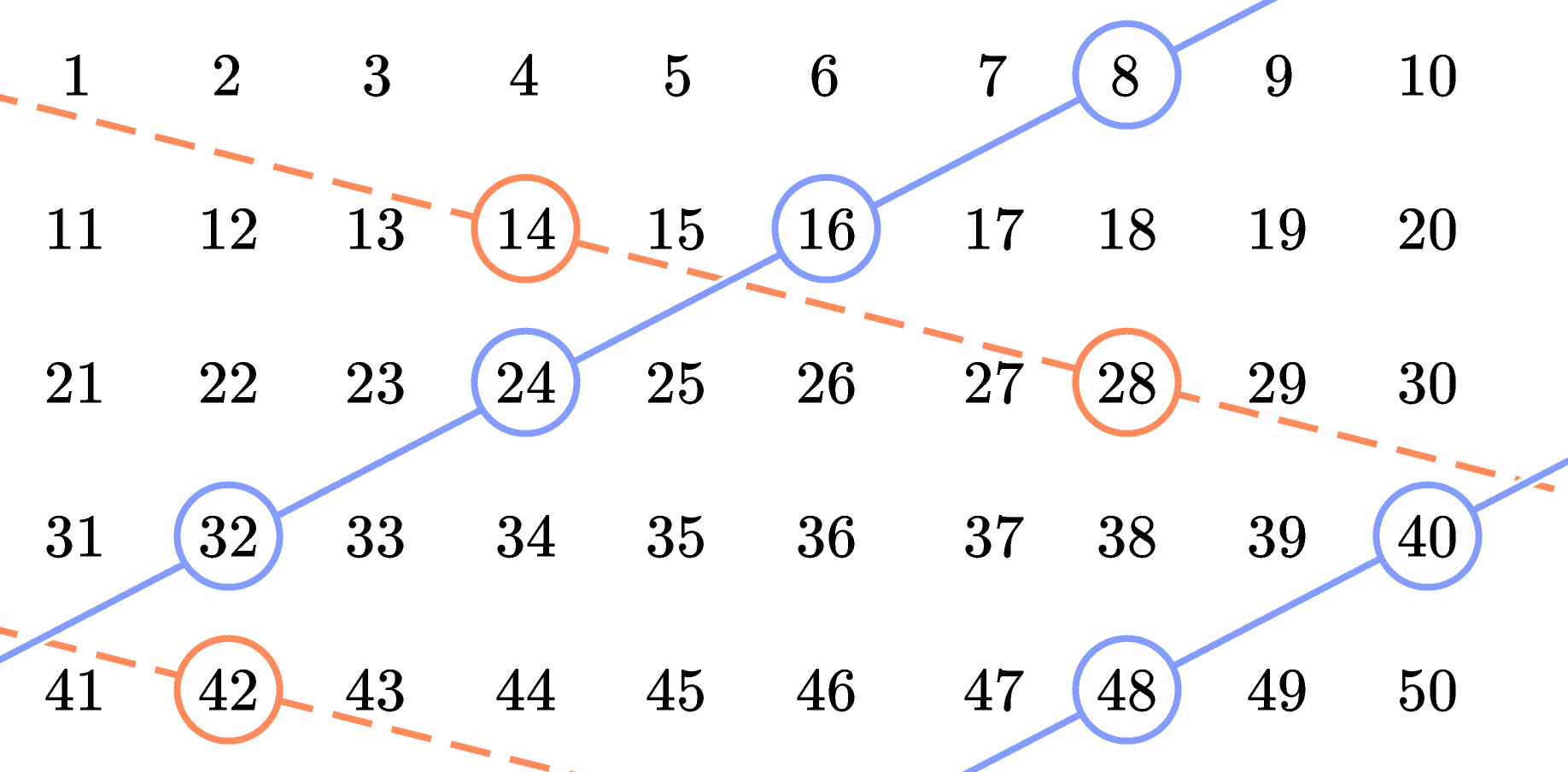We are sunsetting our community features by July 2, 2021. Learn more here.
×

Problem Loading...

Note Loading...

Set Loading...